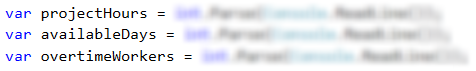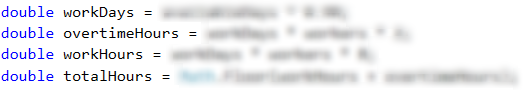# Problem: Firm

A firm gets a request for creating a project for which a certain number of hours are needed. The firm has a certain number of days. During 10% of the days, the workers are being trained and cannot work on the project. A normal working day is 8 hours long. The project is important for the firm and every worker must work on it with overtime of 2 hours per day.

The hours must be rounded down to the nearest integer (for example, 6.98 hours are rounded to 6 hours).

Write a program that calculates whether the firm can finish the project on time and how many hours more are needed or left.

## Input Data

The input data is read from the console and contains exactly three lines:

• On the first line are the needed hoursan integer in the range of [0 … 200 000].
• On the second line are the days that the firm hasan integer in the range of [0 … 20 000].
• On the third line are the number of all workersan integer in the range of [0 … 200].

## Output Data

Print one line on the console:

• If the time is enough:
• "Yes!{the hours left} hours left.".
• If the time is NOT enough:
• "Not enough time!{additional hours} hours needed.".

## Sample Input and Output

Input Output Input Output
90
7
3
Yes!99 hours left. 99
3
1
Not enough time!72 hours needed.

## Hints and Guidelines

In order to solve the problem, we will read the input data. Then, we will write a few conditional statements and do some calculations. Finally, we will print the result.

Firstly, we need to decide what data types we are going to use for the input data.### Auxiliary Calculations

The next step is to calculate the number of total working hours by multiplying the working days by 8 (every working day is 8 hours long) with the number of workers and then sum them with the overtime. The working days equal 90% of the days that the firm has. The overtime equals to the result of the multiplication of the number of workers by 2 (the possible hours of overtime) and then it is multiplied by the number of days that the firm has. From the task requirements we see that the hours should be rounded down to the nearest integer, which we will do with the method Math.Floor(…).### Checking the Conditions and Printing Output Data

After having done the calculations that are needed to find the value of the working hours, now we have to check whether these hours are enough, or some hours are left.

If the time is enough, we print the result that is specified in the task requirements, which in this case is the difference between the working hours and the hours needed for finishing the project.

If the time is not enough, we print the additional hours that are needed for finishing the project. They equal the difference between the hours for the project and the total working hours.